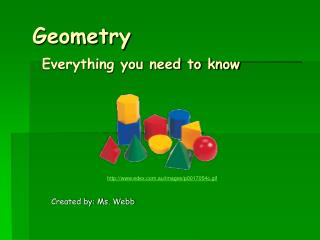DownloadDownload PresentationGeometry Everything you need to know

Geometry Everything you need to know

Télécharger la présentationGeometry Everything you need to know

- - - - - - - - - - - - - - - - - - - - - - - - - - - E N D - - - - - - - - - - - - - - - - - - - - - - - - - - -
Presentation Transcript

1. GeometryEverything you need to know Created by: Ms. Webb http://www.edex.com.au/images/p0017054c.gif

2. What is geometry? • Geometry is the study of solids, surfaces, points, lines, curves and angles and their relationship in space. • Geometry is the mathematics of shapes

3. What are plane figures? • A plane figure is a flat closed figure that is made up of straightorcurved lines. • It can also be made up of straight and curved lines.

4. What are polygons? • A polygon is a closed figure made up of straight line segments.

5. Examples of polygons: • Triangle: • Hexagon: • Octagon: • Pentagon:

6. What are quadrilaterals? • A quadrilateral is a polygon that has 4 sides and 4 angels.

7. Examples of quadrilaterals: • Square: • Rectangle: • Trapezoid: • Parallelogram: • Rhombus

8. What are solid figures? • A solid figure is a shape that has length, width and height. I can hold it in my hand.

9. Examples of solid figures: • Cube: • Cone: • Cylinder: • Sphere:

10. Where can I find geometric shapes in the real world?